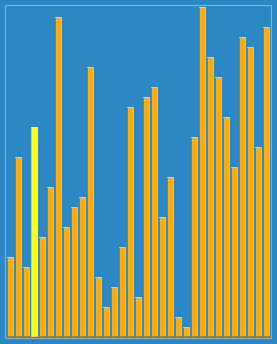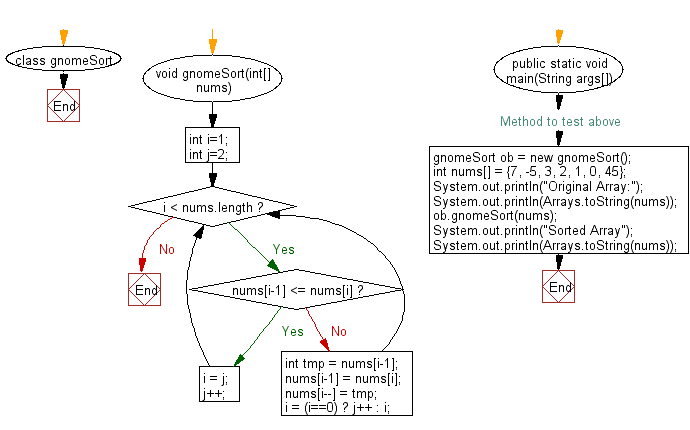﻿ Java exercises: Gnome sort Algorithm - w3resource# Java Exercises: Gnome sort Algorithm

## Java Sorting Algorithm: Exercise-13 with Solution

Write a Java program to sort an array of given integers using Gnome sort Algorithm.

Gnome sort is a sorting algorithm originally proposed by Dr. Hamid Sarbazi-Azad (Professor of Computer Engineering at Sharif University of Technology) in 2000 and called "stupid sort" (not to be confused with bogosort), and then later on described by Dick Grune and named "gnome sort".
The algorithm always finds the first place where two adjacent elements are in the wrong order, and swaps them. It takes advantage of the fact that performing a swap can introduce a new out-of-order adjacent pair only next to the two swapped elements.

A visualization of the gnome sort:Sample Solution:

Java Code:

``````import java.util.Arrays;
class gnomeSort {
void gnomeSort(int[] nums)
{
int i=1;
int j=2;

while(i < nums.length) {
if ( nums[i-1] <= nums[i] )
{
i = j; j++;
} else {
int tmp = nums[i-1];
nums[i-1] = nums[i];
nums[i--] = tmp;
i = (i==0) ? j++ : i;
}
}
}

// Method to test above
public static void main(String args[])
{
gnomeSort ob = new gnomeSort();
int nums[] = {7, -5, 3, 2, 1, 0, 45};
System.out.println("Original Array:");
System.out.println(Arrays.toString(nums));
ob.gnomeSort(nums);
System.out.println("Sorted Array");
System.out.println(Arrays.toString(nums));
}
}
```
```

Sample Output:

```Original Array:
[7, -5, 3, 2, 1, 0, 45]
Sorted Array
[-5, 0, 1, 2, 3, 7, 45]
```

Flowchart:Java Code Editor:

What is the difficulty level of this exercise?

﻿

## Java: Tips of the Day

Array vs ArrayLists:

The main difference between these two is that an Array is of fixed size so once you have created an Array you cannot change it but the ArrayList is not of fixed size. You can create instances of ArrayLists without specifying its size. So if you create such instances of an ArrayList without specifying its size Java will create an instance of an ArrayList of default size.

Once an ArrayList is full it re-sizes itself. In fact, an ArrayList is internally supported by an array. So when an ArrayList is resized it will slow down its performance a bit as the contents of the old Array must be copied to a new Array.

At the same time, it's compulsory to specify the size of an Array directly or indirectly while creating it. And also Arrays can store both primitives and objects while ArrayLists only can store objects.

Ref: https://bit.ly/3o8L2KH